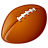# QlikView App Dev

Discussion Board for collaboration related to QlikView App Development.

Announcements
Customer & Partners, DEC. 9, 11 AM ET: Qlik Product & Strategy Roadmap Session: Data Analytics REGISTER NOW
cancel
Showing results for
Did you mean:Creator

## Expression Help - Date

Hi All,

I need some help to excluded dates for my sum function. Currently, I use this formula to exclude name where =”Rick”. Sum({<[Type]={'Cost'},Name-={'Rick'}>}[Amount])”. Instead of the Name field I want to use a “Date” field.

What I want to do is exclude dates where it happen during the quarter. In my dashboard, I have “Reporting Date” field such as 3/31/2017, 6/30/2017, and 9/30/2017. For example: if I selected Reporting Date field 6/30/2017,  I want the formula to exclude “Date” where Date >= 4/1/2017   and Date <=6/30/2017.

Sum({<[Type]={'Cost'},Date >= 4/1/2017   and Date <=6/30/2017>}[Amount])

Any input greatly appreciated!

Many Thanks,

Frank

1 Solution

Accepted SolutionsMaster II

try this

sum({<[Type] = {'Cost'}, Date -= {">=\$(=Date(quarterstart(Max(Date)),'MM/DD/YYYY')) <=\$(=Date(Max(Date),'MM/DD/YYYY'))"}>}[Amount])

Learning never stops.
4 RepliesPartner

Sum({<[Type]={'Cost'},Date >= 4/1/2017   and Date <=6/30/2017>}[Amount])

If here 4/1/2017 is your year start of selected date (6/30/2017) then,

sum({<[Type] = {'Cost'}, Date = {">=\$(=Date(YearStart(Max(Date),0,4),'M/D/YYYY'))<=\$(=Date(Max(Date),'M/D/YYYY'))"}>}[Amount])MVP

In fact, Dates seems static. Would you go same then simply to call. If you notice below statement you could get some minus symbol before equal sign.

Sum({<[Type]={'Cost'},Name-={'Rick'}, Date -= {">=4/1/2017 <=6/30/2017"}>}[Amount])

OR

Sum({<[Type]={'Cost'},Name-={'Rick'}, Date -= {"=Date >= 4/1/2017 and Date <= 6/30/2017"}>}[Amount])

Note - Make sure to clear what you need if dynamic needed.

Before develop something, think If placed (The Right information | To the right people | At the Right time | In the Right place | With the Right context)Master II

try this

sum({<[Type] = {'Cost'}, Date -= {">=\$(=Date(quarterstart(Max(Date)),'MM/DD/YYYY')) <=\$(=Date(Max(Date),'MM/DD/YYYY'))"}>}[Amount])

Learning never stops.Creator
Author

All,

Thanks for quick responses. Will check it out later.Community Browser